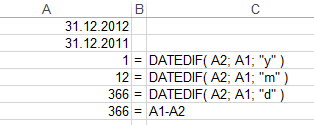﻿ Calculate Age in Excel using DateDif Excel FunctionMicrosoft Office Tutorials and Downloads Microsoft Office Tutorials, Downloads, Tools and Programs like Excel, Word, Outlook, Project, etc.

# Calculate Age in Excel using DateDif Excel Function

This Excel tutorial shows how to calculate age in Excel using DateDif function with changing time interval input variables to get the desired output.

Excel DATEDIF function is used to calculate difference between two dates and express time difference in forms of days, months or years. Since DATEDIF Excel function is an undocumented function, Excel users sometimes use workarounds to calculate age of a person in terms of years, for example. But Excel DateDif date difference function provides a direct use to express time period between two dates in desired time intervals like years, months or days. If you use minus (-) between two dates in an Excel formula, it returns the date difference only in forms of days.

Although calculating time period between two dates is a frequent task and has been implemented using build-in functions in many development languages and programming scripts, Microsoft Excel did not have a date difference function listed in help documentation. It is interesting that DateDif function in Excel is kept undocumented and away from the eyes of Microsoft Excel users.

Let's not make an example how users can use DateDif function in Excel worksheets.
But before keep the syntax of Excel function DateDif in mind:## Calculate Age Function in Excel Worksheet

If your requirement is to express date difference in forms of a combination of different time intervals like 25 years 3 months and 12 days, then you need a more complex formula. This complex date difference formula will use a number of Excel DateDif function to form the desired output.
Let's apply this formula on an Excel age calculation formula to build age function.

Let's assume that you want to calculate the age of a person who was born at 2nd of June in 1995. Write todays date at A1 cell and the birthdate of the person at A2 cell.
Besides the time intervals "y", "m" and "d" we have additional time intervals that can be used with Excel DATEDIFF function.

These additional DateDif intervals are ym, yd and md intervals. These new intervals work like a mod function.

ym interval parameter returns the number of months (m) remaining after all years (y) are excluded.
yd interval parameter returns the number of days (d) remaining after all years (y) are excluded.
md interval parameter returns the number of days (d) remaining after all months (m) are excluded.So we can get compute age of a person in Excel using the below formula:
=DATEDIF(A2;A1;"y")&" years "&DATEDIF(A2;A1;"ym")&" months "&DATEDIF(A2;A1;"md")&" days "

If you are not sure A2 is small date where else A1 cell has bigger date, you can prevent Microsoft Excel to display error using ERRORIF function for error handling.

=IFERROR(DATEDIF(A1;A2;"y");DATEDIF(A2;A1;"y"))&" years "&IFERROR(DATEDIF(A1;A2;"ym");DATEDIF(A2;A1;"ym")&" months "&IFERROR(DATEDIF(A1;A2;"md");DATEDIF(A2;A1;"md")&" days "))